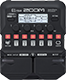# Primeira posição som limpo

Discussion in 'Zoom G1/G1X Four' started by Anderson Scarparo, Apr 10, 2022.

1. Primeira posição som limpoDevice: Zoom G1 Four
Firmware: 2.00

Name on device: PEDAIS 1
Optimized for: Guitar Amp

Effects chain:Primeira posição som limpo com equalizador e noise reduction

Effect: "Gt GEQ 7" (Filter), active - "yes"
"100" = 7.5
"200" = 3.5
"400" = 0.0
"800" = 4.0
"1.6k" = 2.5
"3.2k" = 0.0
"6.4k" = -10.0
"VOL" = 75

Effect: "NoiseGate" (Dynamics), active - "yes"
"Detect" = GTRIN
"Depth" = 92
"Threshold" = 41
"Decay" = 0

Effect: "Phaser" (Modulation), active - "no"
"Color" = 8 STG
"Depth" = 35
"Rate" = 13
"Resonance" = 0

Effect: "VinFLNGR" (Modulation), active - "no"
"PreD" = 25
"Depth" = 47
"Rate" = 7
"Resonance" = 2

Effect: "Delay" (Delay), active - "no"
"Time" = 340
"F.B." = 40
"Mix" = 35
"Tail" = On

Patch Volume: 100